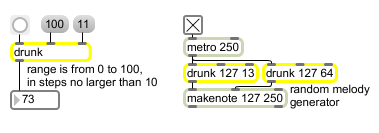# drunk

Output random numbers in a moving range

## Description

drunk will perform a "drunk" walk by outputting random numbers within a moving specified range.

## Arguments

Name Type Opt Description
maximum-value and step-size int opt The first argument sets an initial value for the maximum number which can be output by drunk. The second argument sets an initial limit on the size of random steps taken by drunk; the absolute value of the step size will always be less than the absolute value of this limit. If a negative value is specified as a step size, steps of size zero are never generated. If there are no typed-in arguments, the maximum value is set to 128 and the step size limit is set to 2 (movement up or down by no more than 1).

## Messages

 bang In left inlet: Causes drunk to take a step of random size up or down from its currently stored value. It updates the stored value and sends it out the outlet. int input [int] In left inlet: The number replaces the stored value and is sent out the outlet. (inlet1) maximum [int] In middle inlet: The number is stored as the maximum value that can be output by drunk. (Note: If the specified maximum is less than 0 it is set to 0.) (inlet2) step-size [int] In right inlet: The number limits the step size taken in response to a bang in the left inlet. The step (up or down) will always be less than the absolute value of this number. float input [float] Converted to int. seed random-generator-seed [int] In left inlet: The word seed, followed by a number, "seeds" the drunk object's ran\dom generator, which causes a specific (reproducible) sequence of pseudo-random numbers to occur. The number 0 uses the time elapsed since system startup (an unpredictable value) as the seed, ensuring an unpredictable sequence of numbers. This unpredictable seed is used by default when the drunk object is created. set input [int] In left inlet: The word set, followed by a number, sets the stored value of drunk to that number without triggering output. The stored value is initially set in the center of the total range (1/2 the maximum value).

## Output

int: The number sent out the outlet is automatically limited between 0 and the specified maximum value, and differs from the previously stored number by less than the maximum step size.

## ExamplesNumbers vary aimlessly in small steps taken within the total range